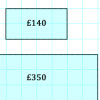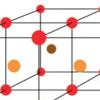Search by Topic

Resources tagged with Creating and manipulating expressions and formulae similar to Number Lines:

Filter by: Content type:
Age range:
Challenge level:

There are 5 results

Broad Topics > Algebraic expressions, equations and formulae > Creating and manipulating expressions and formulaeDiagonal Sums

Age 7 to 11 Challenge Level:

In this 100 square, look at the green square which contains the numbers 2, 3, 12 and 13. What is the sum of the numbers that are diagonally opposite each other? What do you notice?Price Match

Age 7 to 11 Challenge Level:

Can you find pairs of differently sized windows that cost the same?Finding 3D Stacks

Age 7 to 11 Challenge Level:

Can you find a way of counting the spheres in these arrangements?